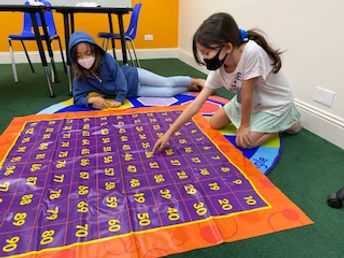## Ms. Dawn

### Target 1​

###### Lesson Type:

New

Number Operation

:

Integer Composition

Recognize a whole number is a multiple of each of its factors.

###### 1:

Determine whether a given whole number in the range of one to 100 is a multiple of a given one-digit number.

###### 2:

Using a hundred’s chart, identify all the multiples of two through ten.

###### 3:

Recognize patterns in multiples, setting the foundation for developing computational fluency in multiplication.

3rd

###### Vocabulary:

Multiple, Factor, Pattern

Activities:

• Students solved a variety of division problems using strategies they've learned. Students chose the strategy that works best for them.

• Students used their knowledge of multiples to build a multiple ladder and then inserted it into a factor rainbow.### Home Exploration

###### Guiding Questions:## Absent Students:

### Target 2

:

###### 1:

Imagine an object being manipulated in space (rotated, folded, cut, etc.)

###### 2:

Explore point of view.

###### 3:

Imagine different visual perspectives.

3rd

###### Vocabulary:

Space, Rotate, Transform, Reasoning, Spatial, 3D, 2D, Diagrams, Visualize

Activities:

• Students determined what 2D pattern created the 3D cube.

• Students identified 3D shapes from a topdown 2D view.### Home Exploration

###### Guiding Questions:### Target 3

:

###### 1:

Understand that dividing numbers makes numbers smaller.

###### 2:

Understand there are multiple ways to divide a multi-digit number.

###### 3:

Understand that division can be represented with repeated subtraction.

###### 4:

Understand that division represents taking a quantity and determining how many equal groups of objects can be made.

4th

###### Vocabulary:

Multi-digit, Repeated subtraction, Quantity, Divisor, Dividend, Quotient

Activities:

• Students used a 3 digit by 1 digit format to solve their long division problems they created using a die.

• Students solved a variety of division problems using strategies they've learned. Students chose the strategy that works best for them.### Home Exploration